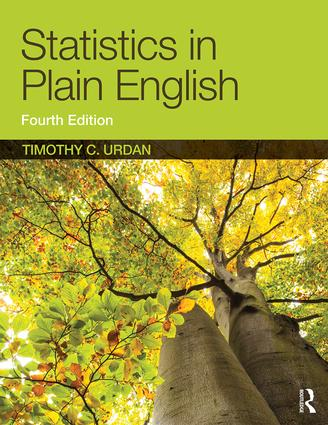Welcome

This is the companion website for Statistics in Plain English by Timothy C. Urdan.

This introductory textbook provides an inexpensive, brief overview of statistics to help readers gain a better understanding of how statistics work and how to interpret them correctly. Each chapter describes a different statistical technique, ranging from basic concepts like central tendency and describing distributions to more advanced concepts such as t tests, regression, repeated measures ANOVA, and factor analysis. Each chapter begins with a short description of the statistic and when it should be used. This is followed by a more in-depth explanation of how the statistic works. Finally, each chapter ends with an example of the statistic in use, and a sample of how the results of analyses using the statistic might be written up for publication. A glossary of statistical terms and symbols is also included. Using the author’s own data and examples from published research and the popular media, the book is a straightforward and accessible guide to statistics.

Here you will find the following resources for students:

• Chapter summaries
• Links to useful tools and resources
• Detailed solutions to the text’s work problems
• Videos showing how to calculate statistics, how to calculate and interpret the appendices, and how to understand some of the more confusing tables of output produced by SPSS
• SPSS datasets

And for instructors and lecturers:

• PowerPoint Lecture Slides
• Test questions